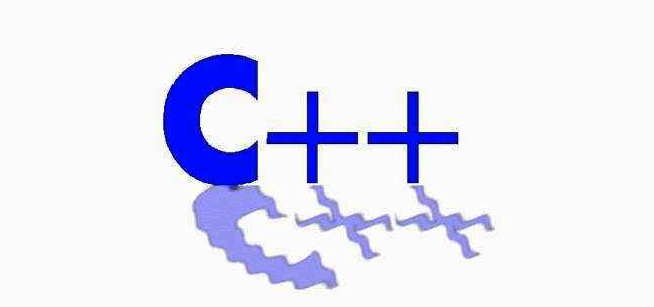## 第四章表达式 [sizeof&强制类型转换]

sizeof运算符:

``````cout << sizeof(1) << endl;
int a[] = {1,2,3};
cout << sizeof(a) << endl; // 12
int *p = a;
cout << sizeof(p) << endl; // 4
``````

const_cast运算符:

``````int i = 1;
int *p = &i;
const int *f = const_cast<const int*>(p); //加上const
*p = 23; // ok
*f = 23; // err

const int g = 1;
int *h = const_cast<int*>(&g);  // 去掉const
*h = 2; // ok
g =2; // err
``````

static_cast运算符:

``````复制代码

char a = 'a';
int b = static_cast<char>(a);// ok

int i = 1;
const int f = static_cast<const int>(i); // ok

const int g = 2;
int *h = static_cast<int*>(&g); // err 不能转换g去掉const

``````

reinterpret_cast&&dynamic_cast:

"三分天下"八九十;